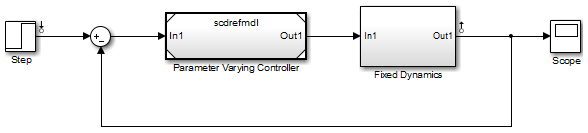# linlftfold

Combine linearization results from specified blocks and model

## Syntax

```lin = linlftfold(lin_fixed,blocksubs) ```

## Description

`lin = linlftfold(lin_fixed,blocksubs)` combines the following linearization results into one linear model `lin`:

• Linear model `lin_fixed`, which does not include the contribution of specified blocks in your Simulink® model.

Compute `lin_fixed` using `linlft`.

• Block linearizations for the blocks excluded from `lin_fixed`

You specify the block linearizations in a structure array `blocksubs`, which contains two fields:

• `'Name'` is a character vector or string specifying the block path of the Simulink block to replace.

• `'Value'` is the value of the linearization for each block.

## Examples

collapse all

In this example, the `scdtopmdl` model contains two subsystems in the feedforward path.

• Fixed portion, which contains everything except the Parameter Varying Controller model reference

• Parameter Varying Controller model, which references the `scdrefmdl` model

Open the top-level model.

```topmdl = 'scdtopmdl'; open_system(topmdl)```Linearize this model without the Parameter Varying Controller block.

```io = getlinio(topmdl); blocks = {'scdtopmdl/Parameter Varying Controller'}; sys_fixed = linlft(topmdl,io,blocks);```

Linearize the controller model.

```refmdl = 'scdrefmdl'; load_system(refmdl); sys_pv = linearize(refmdl);```

Combine the linearization results.

`BlockSubs(1) = struct('Name',blocks{1},'Value',sys_pv);`